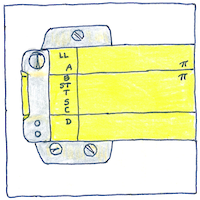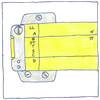# 1614 Logarithm

## The book of science

Tom Sharp

 John Napier mathematics## Logarithm

• The Description of the Wonderful Canon of Logarithms,
• and the use of which not only in
• Trigonometry, but also in all
• Mathematical Calculations, most fully
• and easily explained in the most
• expeditious manner.
• By the author and discoverer
• John Napier.
• *
• Herein providing a method with a table
• to reduce to their logarithms
• numbers that must be multiplied or divided,
• which logarithms can be more expeditiously

## Proportional numbers

• John Napier coined the term.
• Greek for reason or proportion, logos,
• combined with Greek for number, arithmos.
• Today logarithms are defined algebraically;
• however, John Napier defined his numbers geometrically
• so that each decrease is a fixed proportion of the remaining.

## Analogs

• That you may be taught a procedure
• and perform this procedure using a pencil and paper,
• that you may create and manipulate symbols
• that stand for quantities and operations,
• that you may explain the operations and meanings
• so that others may perform similar procedures,
• that you may be taught the use of the same,
• and may create new procedures based on them,
• that your life may have a recognizable pattern
• and by understanding it you may achieve grace,
• is like the transmission of a spiritual truth
• from master to disciple, which no one can do for you.

Decimal arithmetic was explained by Simon Stevin in 1585, but Stevin’s notation, lacking the decimal point, was cumbersome. The decimal point was introduced by Bartholomaeus Pitiscus, then adopted and taught by John Napier. The popularity of logarithms made its use common.

John Napier also invented a calculating aid in 1617 known as “Napier’s bones.” Only five years later, in 1622, William Oughtred created the first slide rule, an analog calculator based on logarithmic scales. Blaise Pascal invented the mechanical calculator, known as the “Pascaline,” in 1645. Charles Babbage’s Difference Engine, in 1822, was a more complex mechanical calculator, but was controlled by programs stored on punch cards, like the first computers.